CBSE Class 10 Sample Paper for 2019 Boards

Class 10
Solutions of Sample Papers for Class 10 Boards

Question 21 (OR 1 st question)

Water is flowing at the rate of 15 km per hour through a pipe of diameter 14cm into a rectangular tank which is 50 m long and 44 m wide. Find the time in which the level of water in the tank will rise by 21 cm.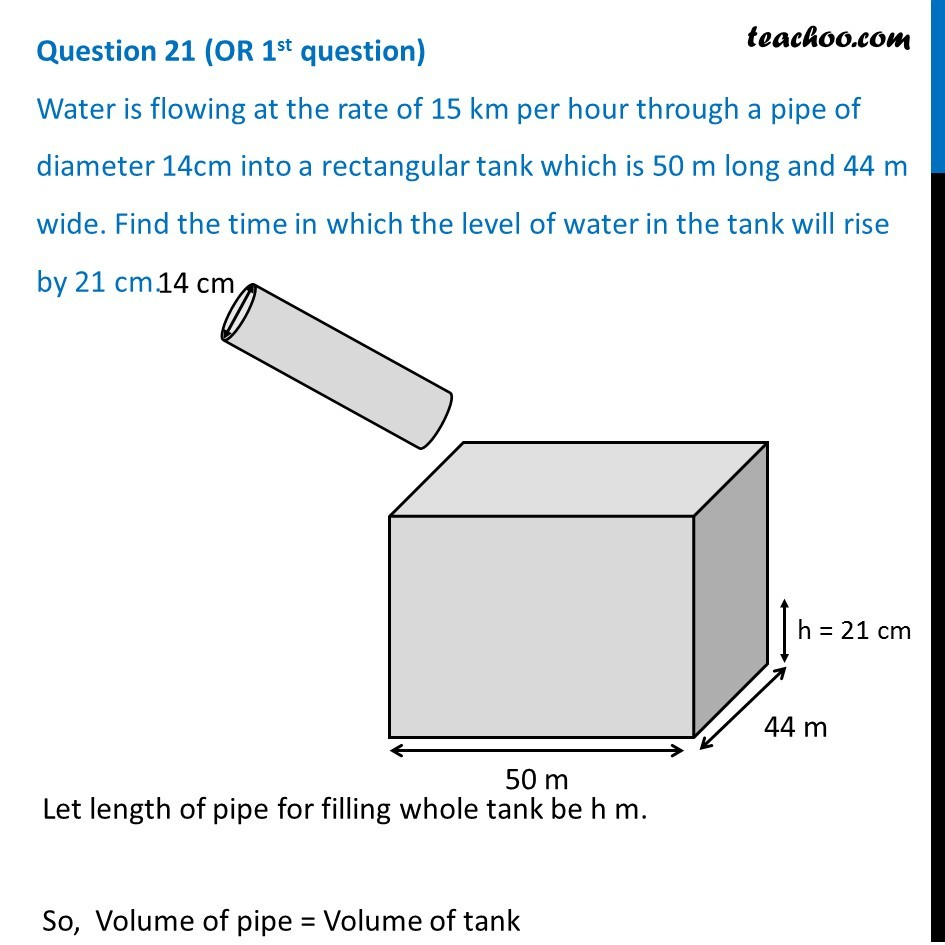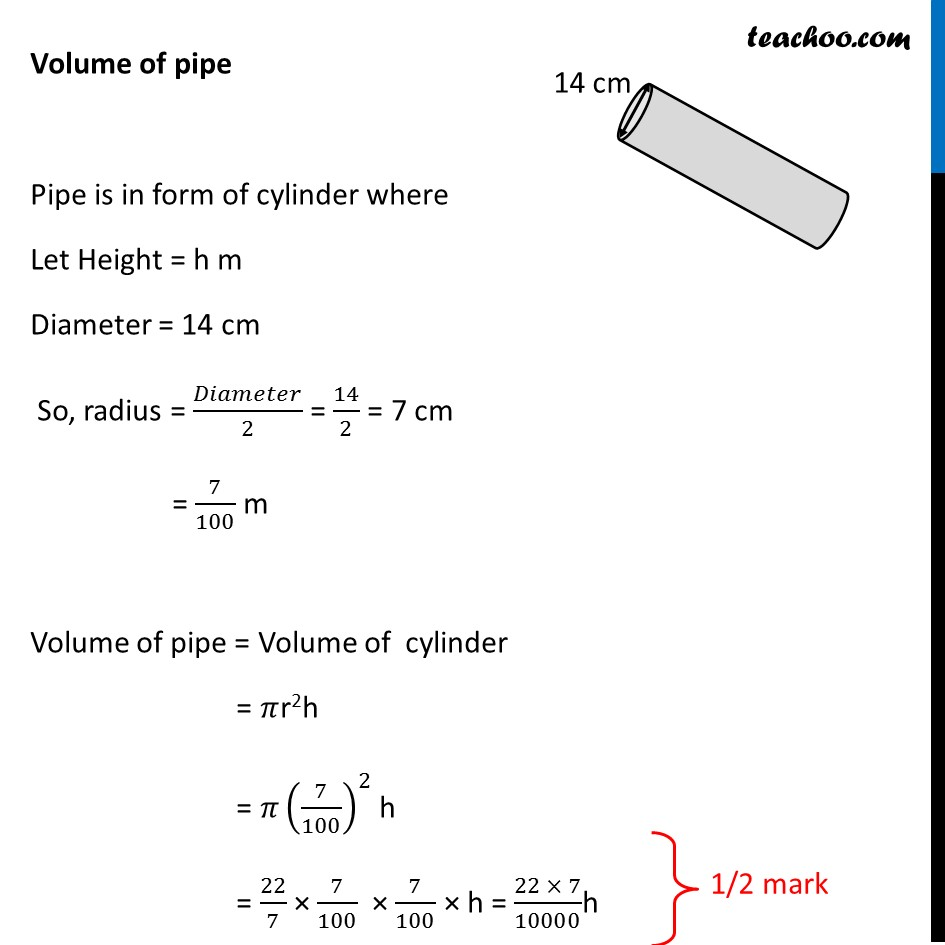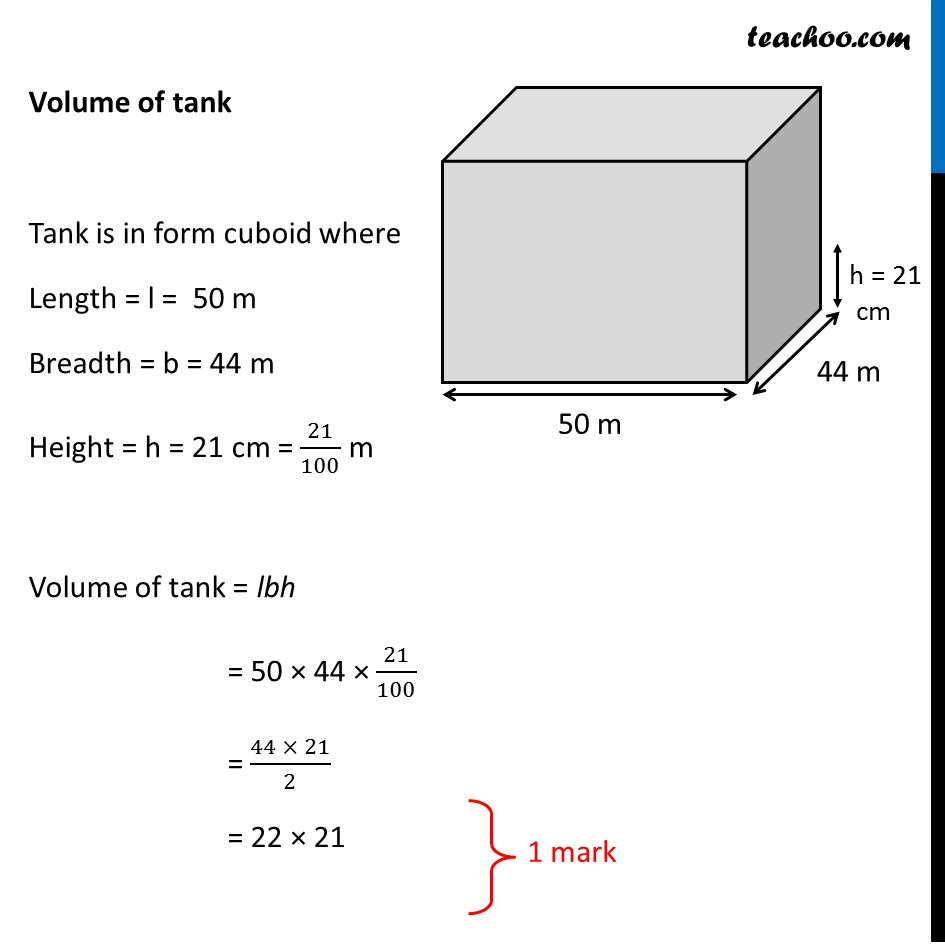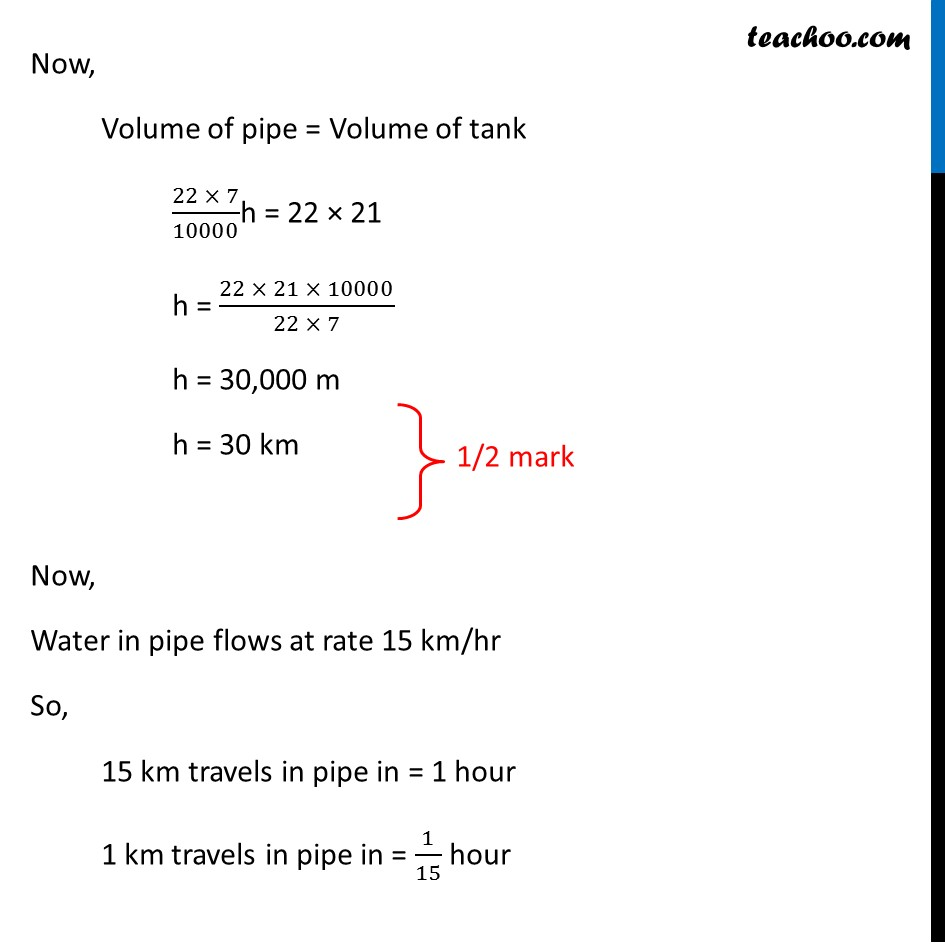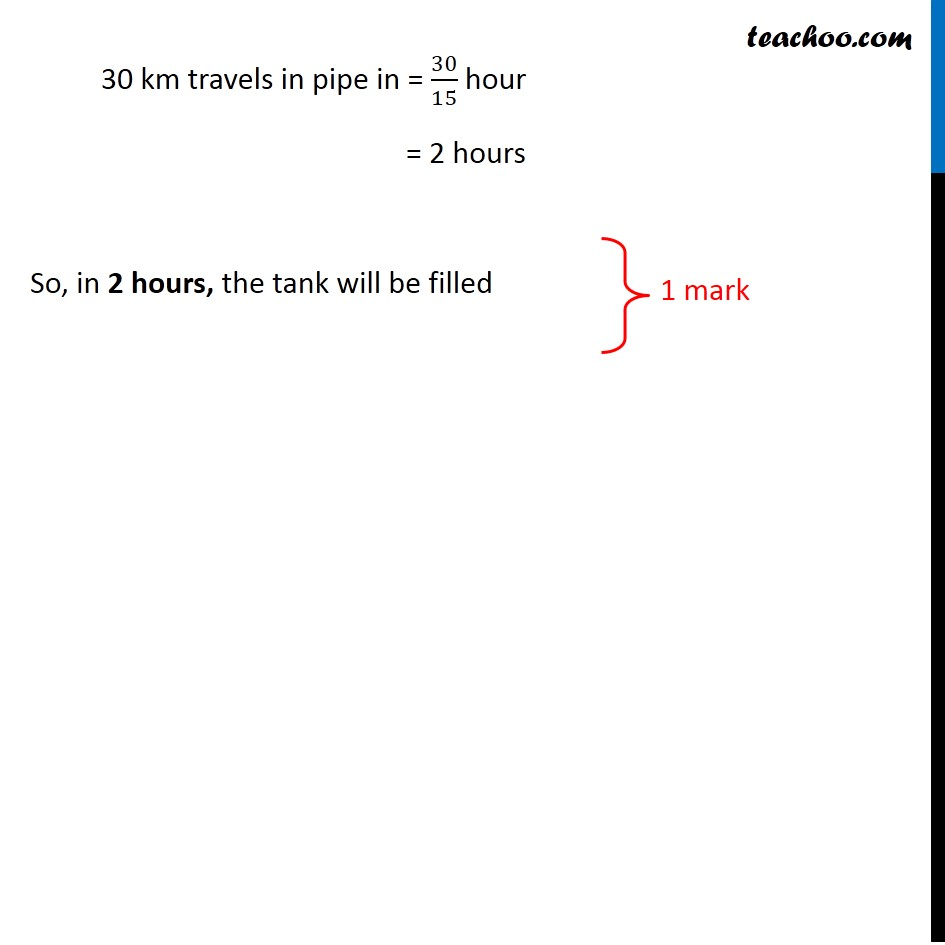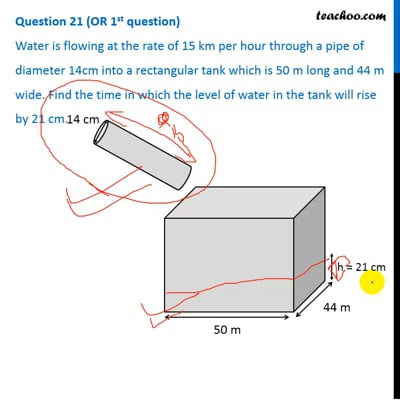This video is only available for Teachoo black users

Get live Maths 1-on-1 Classs - Class 6 to 12

### Transcript

Question 21 (OR 1st question) Water is flowing at the rate of 15 km per hour through a pipe of diameter 14cm into a rectangular tank which is 50 m long and 44 m wide. Find the time in which the level of water in the tank will rise by 21 cm. Let length of pipe for filling whole tank be h m. So, Volume of pipe = Volume of tank Volume of pipe Pipe is in form of cylinder where Let Height = h m Diameter = 14 cm So, radius = 𝐷𝑖𝑎𝑚𝑒𝑡𝑒𝑟/2 = 14/2 = 7 cm = 7/100 m Volume of pipe = Volume of cylinder = 𝜋r2h = 𝜋(7/100)^2 h = 22/7 × 7/100 × 7/100 × h = (22 × 7)/10000h Volume of tank Tank is in form cuboid where Length = l = 50 m Breadth = b = 44 m Height = h = 21 cm = 21/100 m Volume of tank = lbh = 50 × 44 × 21/100 = (44\ × 21)/2 = 22 × 21 Now, Volume of pipe = Volume of tank (22 × 7)/10000h = 22 × 21 h = (22 × 21 × 10000)/(22 × 7) h = 30,000 m h = 30 km Now, Water in pipe flows at rate 15 km/hr So, 15 km travels in pipe in = 1 hour 1 km travels in pipe in = 1/15 hour 30 km travels in pipe in = 30/15 hour = 2 hours So, in 2 hours, the tank will be filled